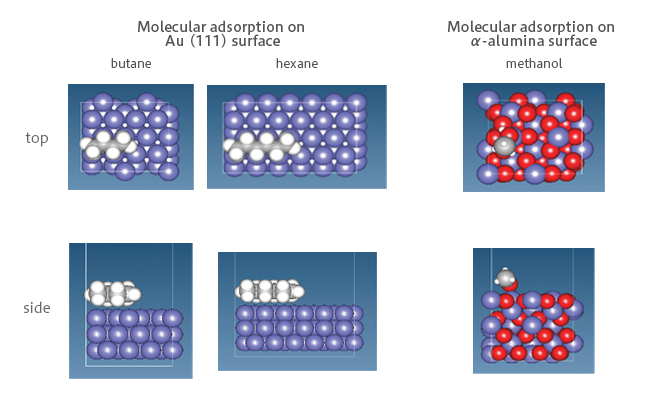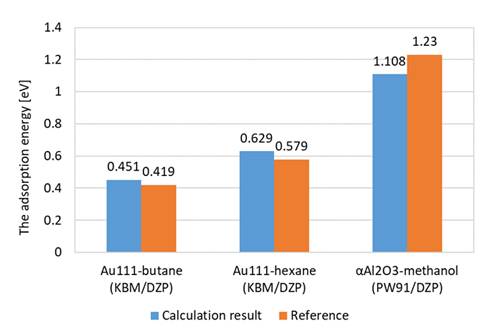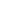Quantum Chemistry / DFT
Small molecule penetration / diffusion / adsorption
Interface / Phase Separation / Particle Dispersion
Materials Science

# The adsorption energy of molecules on solid surfaces

## Purpose and Method

The adsorption energy, which is the change in energy when a molecule is adsorbed on a solid surface, is one of the most basic parameters for understanding the behavior of a molecule on a surface, and affects the adhesion phenomenon, wettability, and dispersibility of particles.

In this case study, the adsorption energies of molecules on Au (111) surface and α-alumina surface were calculated by SIESTA using density functional theory.

In SIESTA, since the Basis Set Superposition Error (BSSE) is included in the calculation of the interaction energy, the adsorption energy is calculated with the formula below:

$$\Delta E^{\rm \scriptsize CP}_{ads}$$ = $$\Delta E^{\rm \scriptsize CP}_{\rm surface-mol}$$ + $$\Delta E_{\rm surface, deform}$$ + $$\Delta E_{\rm mol, deform}$$

$$\Delta E^{\rm \scriptsize CP}_{\rm surface-mol}$$:The interaction energy between the surface and the molecule corrected by the Counterpoise method

$$\Delta E_{\rm surface, deform}$$:The energy difference between the adsorbed and isolated states of the surface

$$\Delta E_{\rm mol, deform}$$:The energy difference between the adsorbed and isolated states of the moleculeFigure 1　The calculation model of adsorption energy for SIESTA

## Simulation result

Adsorption energies of alkane molecules on Au(111) were calculated using KBM functional, which takes into account van der Waals interaction. Obtained values are almost equivalent as the reference values . For the α-alumina surface, calculation was performed by applying PW91 functional with according to the reference . As a result, we obtained the result with an error of 0.1 eV. These results show that accurate calculations could be performed by SIESTA. (Figure 2)Figure2　Comparison of calculation results of adsorption energy by SIESTA with references

• Reference
•  Wetterer, S. M.; Lavrich, D. J.; Cummings, T.; Bernasek, S. L.;Scoles, G. J. Phys. Chem. 1998, 102, 9266
 Ø. Borck and E. Schröder, J. Phys. Condens. Matter, vol. 18, 1–12, 2006.This website uses cookies to improve functionality and performance. If you continue browsing the site, you are giving implied consent to the use of cookies on this website. If you want to know more or refuse consent, read our Cookie Policy.

Accept